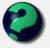# Math Is Fun Forum

Discussion about math, puzzles, games and fun.   Useful symbols: ÷ × ½ √ ∞ ≠ ≤ ≥ ≈ ⇒ ± ∈ Δ θ ∴ ∑ ∫  π  -¹ ² ³ °

You are not logged in.

## #1 2005-06-22 09:55:09

NIH
MemberRegistered: 2005-06-14
Posts: 33

### Equilateral triangles

Here's a cool fact about equilateral triangles.

Draw an equilateral triangle.  Pick a point inside the triangle.  Now calculate (or measure) the perpendicular distance from the point to each side of the triangle.  (The perpendicular distance is the distance along a line which is perpendicular to the side; it's the shortest distance from the point to the side.)  Add up the three distances.  Then pick another point, and do the same.  You should get the same sum!

The sum of the perpendicular distances from any point inside a given equilateral triangle to the three sides is a constant.

2 + 2 = 5, for large values of 2.

Offline

## #2 2005-06-22 11:17:03

MathsIsFunRegistered: 2005-01-21
Posts: 7,711

### Re: Equilateral triangles

Good one, NIH! That sum *should* be equal to the height of the triangle, am I right?

You know, we could have a few pages called "Fun Facts about Triangles", where we could illustrate these ...

"The physicists defer only to mathematicians, and the mathematicians defer only to God ..."  - Leon M. Lederman

Offline

## #3 2005-06-22 11:38:44

NIH
MemberRegistered: 2005-06-14
Posts: 33

### Re: Equilateral triangles

MathsIsFun wrote:

Good one, NIH! That sum *should* be equal to the height of the triangle, am I right?

Indeed it should!

2 + 2 = 5, for large values of 2.

Offline

## #4 2005-06-24 04:04:20

mathsyperson
ModeratorRegistered: 2005-06-22
Posts: 4,900

### Re: Equilateral triangles

Similar to NIH's thing, pick a point on the equilateral triangle and draw a line parallel to the side adjacent clockwise to the side that you are drawing the line to. (Complicated description, hope you can decode that!) Do the same thing for the other two, and the sum of the lengths of the lines is equal to the length of one of the sides. You can draw lines parallel to the anticlockwise side instead and it'll still work.

Why did the vector cross the road?
It wanted to be normal.

Offline

## #5 2005-06-24 10:56:37

MathsIsFunRegistered: 2005-01-21
Posts: 7,711

### Re: Equilateral triangles

NOW I understand NIH's avatar !

(BTW good effort at describing, mathsy, but I need a picture I think)

"The physicists defer only to mathematicians, and the mathematicians defer only to God ..."  - Leon M. Lederman

Offline

## #6 2005-06-24 20:29:02

mathsyperson
ModeratorRegistered: 2005-06-22
Posts: 4,900

### Re: Equilateral triangles

Here :
^
/    \
/       /\
/       /    \
/ -----X       \
/           \        \
/               \        \
/                   \        \
/                       \        \
-----------------------------

Hmm... not the best picture ever, but does it help?
Also, what do you NOW understand about NIH's avatar?

Last edited by MathsIsFun (2005-06-24 20:44:22)

Why did the vector cross the road?
It wanted to be normal.

Offline

## #7 2005-06-24 20:48:23

MathsIsFunRegistered: 2005-01-21
Posts: 7,711

### Re: Equilateral triangles

Nice diagram ... hmmm ... I will have to check to see if that is right *reaches for pen and paper*

The reference to NIH's logo? Running around the inside of a square - or triangle!

"The physicists defer only to mathematicians, and the mathematicians defer only to God ..."  - Leon M. Lederman

Offline

## #8 2005-06-24 20:49:39

Roraborealis
MemberRegistered: 2005-03-17
Posts: 1,594

### Re: Equilateral triangles

mathsyperson wrote:

Also, what do you NOW understand about NIH's avatar?

That I once had that avatar.

School is practice for the future. Practice makes perfect. But - nobody's perfect, so why practice?

Offline